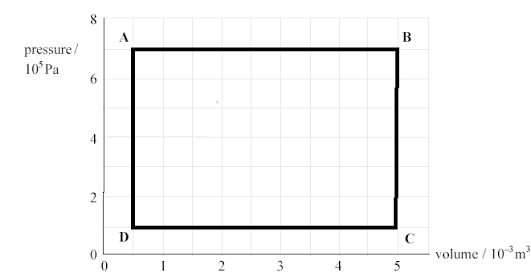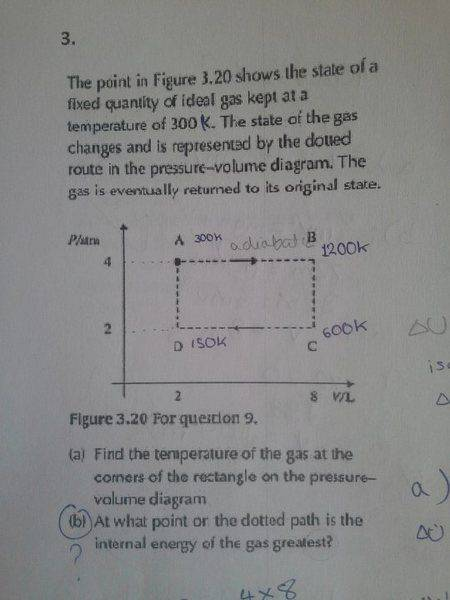# Pressure-volume graph and internal energy

• NihalRi
In summary, the conversation discusses a pressure volume graph with a gas that forms a rectangle, labeled A to D. The question is at what point or line is the internal energy of the gas greatest. It is noted that the temperature increases from A to B, which is strange because it is thought that with expansion, the internal energy decreases. However, it is clarified that the process is going in a clockwise direction and that the temperature is increasing along AD. It is also mentioned that work is done by the gas, leading to a decrease in work and an increase in temperature, thus increasing the internal energy. Finally, it is noted that Q needs to be added to the system for this to happen.

## Homework Statement

There is a pressure volume graph with the gas changes shown, forming a rectangle. The corners are labled A to D starting from the upper left corner heading on to the right until it returns to point A again.
The question is at what point, or line is the internal energy of the gas greatest?Like this, except the numbers are different.

ΔU = Q-W

## The Attempt at a Solution

The prior question asked to find the temperatures at each point provided A was at 300K.
So I thought that the internal energy of the gas would be maximum when it's at low volume and high pressure, therefore at point A. But at B & C it is hotter meaning it must have gained energy from an external source. So going to B it has expanded and gained energy. But by expanding it does work loosing internal energy right? So how come its temperature got higher? Honestly these graphs just confuse me a lot, I'd really appreciate any help.

From A to B the gas is expanding, but the pressure is kept constant.
Assuming the amount of gas stays the same how can this be achieved?

•NihalRi
andrevdh said:
From A to B the gas is expanding, but the pressure is kept constant.
Assuming the amount of gas stays the same how can this be achieved?
The temperature increases. Which means the internal energy increased? Which is strange cause I thought that with expansion the internal energy decreased because work is done by the gas:/

NihalRi said:
The temperature increases. Which means the internal energy increased? Which is strange cause I thought that with expansion the internal energy decreased because work is done by the gas:/
This problem statement fails to tell you whether the process is reversible or irreversible, or whether the process is carried out clockwise or counter-clockwise. The process from A to B can be carried out adiabatically and irreversibly at constant pressure, in which case the temperature at B would be lower than at A. So apparently they meant for the expansion to be reversible.

•NihalRi
Chestermiller said:
This problem statement fails to tell you whether the process is reversible or irreversible, or whether the process is carried out clockwise or counter-clockwise. The process from A to B can be carried out adiabatically and irreversibly at constant pressure, in which case the temperature at B would be lower than at A. So apparently they meant for the expansion to be reversible.
My mistake, I didn't mention that the entire process is going in a clockwise direction. So that means that A to B is adiabatic? and since Q doesn't change the internal energy decreases?

NihalRi said:
My mistake, I didn't mention that the entire process is going in a clockwise direction. So that means that A to B is adiabatic? and since Q doesn't change the internal energy decreases?
It doesn't mean that A to B is adiabatic. If the process is reversible, then, from the ideal gas law, AB has to be isothermal. And, from the ideal gas law, the temperature has to be decreasing along BC. And, from the ideal gas law, CD must be isothermal (but at the lower temperature). And, from the ideal gas law, the temperature has to be increasing along AD. So, where do you think the highest internal energy is?

•NihalRi
If A-B is isothermal , the internal energy wouldn't change thus I think that's where it would be highest. But if these changes were isothermal wouldn't the graph be curved?

NihalRi said:
If A-B is isothermal , the internal energy wouldn't change thus I think that's where it would be highest. But if these changes were isothermal wouldn't the graph be curved?
I'm sorry. I messed up. If the pressure is constant along AB, the ideal gas tells us that the temperature would have to be increasing from A to B. If the volume were held constant along BC, the ideal gas law tells us that the temperature would have to be decreasing along BC. If the pressure were held constant along CD, the ideal gas law tells us that the temperature would have to be decreasing along CD. If the volume is held constant long DA, the ideal gas law tells us that the temperature would have to be increasing along DA.

•NihalRi
Chestermiller said:
I'm sorry. I messed up. If the pressure is constant along AB, the ideal gas tells us that the temperature would have to be increasing from A to B. If the volume were held constant along BC, the ideal gas law tells us that the temperature would have to be decreasing along BC. If the pressure were held constant along CD, the ideal gas law tells us that the temperature would have to be decreasing along CD. If the volume is held constant long DA, the ideal gas law tells us that the temperature would have to be increasing along DA.
Its ok, I think I get it. So the greatest temperature which is at B corespond with the highest internal energy. first an amendment,

ΔU=Q+W (U-internal energy, Q-energy & W-work)

Work is done by the gas meaning W decreases, but there is an increase in temperature leading the internal energy to increase, but for that to happen Q needed to be added to the system. phew
I think I might have overcomplicated the whole thing, this whole time I had overlooked the fact that Q had to be added:) that made it look like the equation contradicted itself:)

Thank you

NihalRi said:
Its ok, I think I get it. So the greatest temperature which is at B corespond with the highest internal energy. first an amendment,

ΔU=Q+W (U-internal energy, Q-energy & W-work)

Work is done by the gas meaning W decreases, but there is an increase in temperature leading the internal energy to increase, but for that to happen Q needed to be added to the system. phew
I think I might have overcomplicated the whole thing, this whole time I had overlooked the fact that Q had to be added:) that made it look like the equation contradicted itself:)

Thank you
In the version of the 1st law that you wrote, W is the work done on the gas. If W is the work done by the gas, then ΔU=Q-W.

•NihalRi
The gas does positive work in expanding from A to B and thus its internal energy decreases, but since its temperature is increased it also gains energy from this process. The difference between the heat gained and the work done by the gas is the change in its internal energy.

•NihalRi
andrevdh said:
The gas does positive work in expanding from A to B and thus its internal energy decreases, but since its temperature is increased it also gains energy from this process. The difference between the heat gained and the work done by the gas is the change in its internal energy.
The wording of this is very confusing. "The gas does positive work in expanding from A to B and thus its internal energy decreases". I think what you mean is that "The gas does positive work in expanding from A to B and thus its internal energy tends to decrease, but this is more than offset by the absorption of heat from the surroundings."

andrevdh said:
The gas does positive work in expanding from A to B and thus its internal energy decreases, but since its temperature is increased it also gains energy from this process. The difference between the heat gained and the work done by the gas is the change in its internal energy.
Can the energy gained and change in internal energy be equal to the work done by the gas? I think that's what happens in this case because the gas only could do an amount of work(expand) equal to the amount of energy it is supplied. The energy added increases the temperature of the gas forcing it to expand in order to maintain the same pressure.

•Chestermiller
Could you maybe post a copy or scan of the original question?Part b

The temperature of a gas is a direct indicator of its internal energy. Increasing its internal energy shows up as an increase in its temperature. So doing work on the gas changes the thermal motion of the gas which registers as an change in its internal energy, the same goes for heat. The energy is thus stored in the termal motion of the molecules and determines the potentiality of the gas to do work say like a steam engine.

Last edited:
•NihalRi
all you need to do is apply the gas law PV =constxT.
From A to B the pressure is constant (isobaric) and therefore increasing the volume by a factor of 4 means trhat the temp increases by a factor of 4 to 1200K...your answer.
in a similar way you have the correct temperatures at points C and D. none of the changes are isothermal or adiabatic !
Work is done BY the gas going from A to B. Change pressure to pascals and volume to m3 and the work done is the area under the line AB .
No work is done from B to C or from D to A
from C to D work is done ON the gas...the area under the line CD
The net work done BY the gas is the area of the loop ABCD
The max internal energy is at the max temp...point B